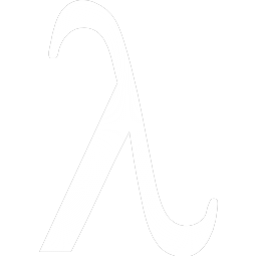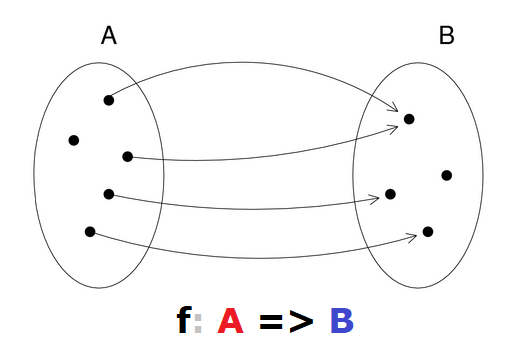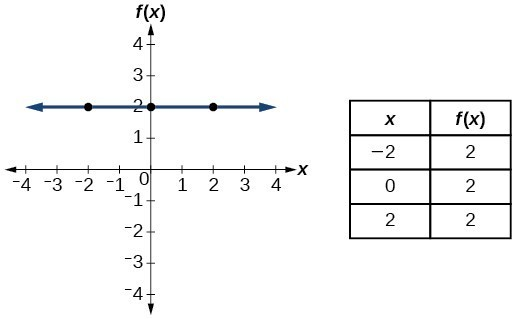# FP Intro## Who am I?

• Full stack(overflow) developer @ OliveBH
• Visit sake.ba for more cool stuff
• Check out hepek static site generator

• immutability
• expressions
• types*
• functions

## Immutability?

``````
var y = 5
y = y + 1           // y-y=1 -> 0=1 😂 WTF?
obj.setStuff(666)

val x = 5
val y = x + 1
val newObj = oldObj.copy(a = 42)
``````

DO NOT CHANGE ANYTHING!
Just create new things based on old things

## Expressions?

``````
/* statement, imperative, NOPE! */
var period = null
if(hours < 12) period = "AM"
else           period = "PM"

/* expression, declarative, YEP! */
val period =
if(hours < 12) "AM" else "PM"

// let period = (hours < 12) ? "AM" : "PM"
// in Scala you can have else-if etc :)
``````

Expression - stuff that returns a value

## Types?

Set of possible values. Also a constraint!

 Byte { -128, .., -1, 0, .., 127 } String all perms of characters.. enum Stuff { A, B } these 2 values interface Shape Shape & implementations

Abuse compiler as much as possible!

## Functions?

``````
// method, NOT first-class value
def formatNumber(x: Int): String = "number: " + x

// lambda, first-class function value/object
val formatNumber = (x: Int) => "number: " + x

formatNumber(3) // number: 3
``````It's a mapping between two types (set of pairs (A, B)).
For every value in A there is a value in B (not necessarily different!)

## WHY THE TROUBLE?

• do one thing* and do it well! (Unix philosophy)
• easier to understand
• explicit dependencies -> easier reusability
• composable, "piping"
• humans can (visually) track only 7 moving objects
...you get the point

Composition!?

Return type of f1 must be input type of f2
(pipes must be compatible)

Composition, again!?

## Higher order functions

Functions that take functions as parameters

``````
val numbers    = List(1, 2, 3)
val numbersInc = numbers.map(n => n + 1)
// numbers.map(_ + 1)
// for(n <- numbers) yield n + 1

// imperative, in-place change... (destructive)
val numbers = ListBuffer(1, 2, 3)
for((n, i) <- numbers.zipWithIndex)
numbers(i) += 1
``````

Say WHAT, not HOW!

`i<list.length` or `i<=list.length` ???
Hmmm, why would I spend my time on that!!?

## Algebraic Data Types

sealed hierarchy (like enums), exhaustive matching (no need for default case)

Option, Either, List ...

### Performance: mutability (if you KNOW what you're doing)

``````
def sumPositive(nums: List[Int]) = {
var total = 0
var i = 0
while(i < nums.size) {
i += 1
if(nums(i) >= 0)
total += nums(i)
}
total
}
``````

Bug at: `i += 1` ... IndexOutOfBoundsException 😦

``````
def sumPositive(nums: List[Int]) = {
nums.filter(_ >= 0).sum
// nums.filter(_ >= 0).fold(0)(_ + _)
}
``````

### Performance: tail recursion

``````
@tailrec
def factorial(n: Int, accum: Int = 1): Int = {
if (n == 0) accum
else factorial(n - 1, n * accum)
}

val fact5 = factorial(5)
``````

Recursion becomes simple
(and efficient!) while loop

No stackoverflow error! :)

## Final thoughts

• write simple, understandable code
• don't use mutability if you don't really need it, narrow its use
• don't use abstractions you don't understand, cause they're cool/popular/everyone's doing it
• everything written in FP style can be written in OOP and imperative style!
(Turing machine and Lambda calculus are equivalent...)

## That's all folks!

### Questions?

What if there's a missing line? Partial function! Boom, a problem!? :D

``````
val divide = (x: Double, y: Double) =>
x / y
``````

We say this funcition is not total.

Throws exception when `y=0`
(FP hates exceptions)

## You're a curious one, aren't you?

• lazy vals - don't compute until really needed (infinite lists? 😮)
• generics - write one sort function, parametrize with comparator
• currying - cool, but not as useful...
• for comprehensions - map, flatMap, foreach (Monads??? OMG NO 😨)
• pattern matching, ADTs - disassemble objects/structures